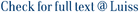This paper deals with a non-standard infinite dimensional linear quadratic control problem arising in the physics of non-stationary states (see, for example, Bertini et al. J Statist Phys 116:831â841, 2004): finding the minimum energy to drive a fixed stationary state xÂ¯ = 0 into an arbitrary non-stationary state x. The Riccati equation (RE) associated with this problem is not standard since the sign of the linear part is opposite to the usual one, thus preventing the use of the known theory. Here we consider the finite horizon case when the leading semigroup is exponentially stable. We prove that the linear selfadjoint operator P(t), associated with the value function, solves the above-mentioned RE (Theorem 4.12). Uniqueness does not hold in general, but we are able to prove a partial uniqueness result in the class of invertible operators (Theorem 4.13). In the special case where the involved operators commute, a more detailed analysis of the set of solutions is given (Theorems 4.14, 4.15 and 4.16). Examples of applications are given.

Minimum energy for linear systems with finite horizon: a non-standard Riccati equation / Acquistapace, P.; Gozzi, F.. - In: MATHEMATICS OF CONTROL SIGNALS AND SYSTEMS. - ISSN 0932-4194. - 29:4(2017), pp. 1-47. [10.1007/s00498-017-0204-y]

### Minimum energy for linear systems with finite horizon: a non-standard Riccati equation

#### Abstract

This paper deals with a non-standard infinite dimensional linear quadratic control problem arising in the physics of non-stationary states (see, for example, Bertini et al. J Statist Phys 116:831â841, 2004): finding the minimum energy to drive a fixed stationary state xÂ¯ = 0 into an arbitrary non-stationary state x. The Riccati equation (RE) associated with this problem is not standard since the sign of the linear part is opposite to the usual one, thus preventing the use of the known theory. Here we consider the finite horizon case when the leading semigroup is exponentially stable. We prove that the linear selfadjoint operator P(t), associated with the value function, solves the above-mentioned RE (Theorem 4.12). Uniqueness does not hold in general, but we are able to prove a partial uniqueness result in the class of invertible operators (Theorem 4.13). In the special case where the involved operators commute, a more detailed analysis of the set of solutions is given (Theorems 4.14, 4.15 and 4.16). Examples of applications are given.
##### Scheda breve Scheda completa Scheda completa (DC)Infinite dimension; Lyapunov equation; Minimum energy; Null controllability; Riccati equation; Value function; Control and Systems Engineering; Signal Processing; Control and Optimization; Applied Mathematics
Minimum energy for linear systems with finite horizon: a non-standard Riccati equation / Acquistapace, P.; Gozzi, F.. - In: MATHEMATICS OF CONTROL SIGNALS AND SYSTEMS. - ISSN 0932-4194. - 29:4(2017), pp. 1-47. [10.1007/s00498-017-0204-y]
File in questo prodotto:
File
AGMCSS2017.pdf

Solo gestori archivio

Tipologia: Documento in Pre-print
Licenza: Tutti i diritti riservati
Dimensione 365.54 kB
Utilizza questo identificativo per citare o creare un link a questo documento: `http://hdl.handle.net/11385/178828`
•1
•1